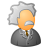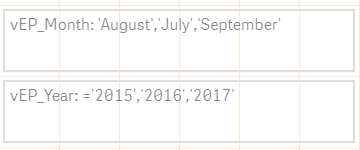Announcements
The way to achieve your own success is the willingness to help somebody else. Go for it!
cancel
Showing results for
Did you mean:Contributor III

## Set Analysis Expression Syntax Issue

I am trying to create a dynamic search string in the set analysis expression using variables, but it doesn't seem to work.

I have 2 variables that store the dynamic selections from year and month field, which are then used in the main expression's set analysis.

Variable Definitions:

Let vEP_Month = Chr(39) & Concat(Distinct If(GetSelectedCount(Revenue_Month) = 0, MaxMonthTxt, Revenue_Month) , Chr(39) &  ',' & Chr(39))  & Chr(39);

Let vEP_Year = Chr(39) & Concat(Distinct  If(GetSelectedCount(Fiscal_Year) = 0, \$(MaxYear), Fiscal_Year), Chr(39) & ',' & Chr(39)) & Chr(39);

Variable Outputs:Expression to calculate Revenue

Sum({<Fiscal_Year = {\$(vEP_Year)}, Revenue_Month = {\$(vEP_Month)}>} Net_Revenue_YTD)MVP

Try this:

Sum({<Fiscal_Year = {\$(=vEP_Year)}, Revenue_Month = {\$(=vEP_Month)}>} Net_Revenue_YTD)

or

Sum({<Fiscal_Year = {\$(=\$(vEP_Year))}, Revenue_Month = {\$(=\$(vEP_Month))}>} Net_Revenue_YTD)Community Browser